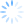In the previous example the “Invoice” object is responsible to retrieve all the values needed to calculate the balance and it also contains the business logic that performs the calculation. This violates the SRP (Single Responsibility Principle). Because of this flaw when we test the calculation of the Balance we need to inject a test double so that the invoice can retrieve values. Why should we need to pass a Service when the only thing our Invoice should be responsible for is the calculation of the Balance?

In the code example here below we specialized the Invoice class. Only what is directly needed is passed in: the input data to calculate the balance. To adhere to the SRP we extract the logic that retrieves the data from our DataLayer out of the Invoice class and create a Repository class (see Repository pattern). We don’t need any mock object anymore what enhance the readability and robustness of our test. By adhering to the SRP we improve our design and enable for better testability.

SUT

`public class Invoice`
`{`
`    private int _balance;`
` `
`    public Invoice(MeteringValues[] dailyValues, int offPeakPrice, int peakPrice, int advances)`
`    {`
` `
`        int peakConsumption = CalculatePeakConsumtion(dailyValues);`
`        int offPeakConsumtion = CalculateOffPeakConsumtion(dailyValues);`
` `
`        _balance = CalculateBalance(`
`                            peakConsumption, `
`                            peakPrice, `
`                            offPeakConsumtion, `
`                            offPeakPrice, `
`                            advances`
`                            );`
`    }`
` `
`...`
`}`
` `
`public class InvoiceRepository`
`{`
`    private IDataLayer _db;`
`    public InvoiceRepository(IDataLayer db)`
`    {`
`        _db = db;`
`    }`
`    public Invoice GetInvoice(int clientID)`
`    {`
`        MeteringValues[] dailyValues = _db.GetMeteringValues(clientID);`
`        int offPeakPrice = _db.GetOffPeakPrice();`
`        int peakPrice = _db.GetPeakPrice();`
`        int advances = _db.GetAdvances(clientID);`
` `
`        return new Invoice(dailyValues, offPeakPrice, peakPrice, advances);`
`    }`
`}`